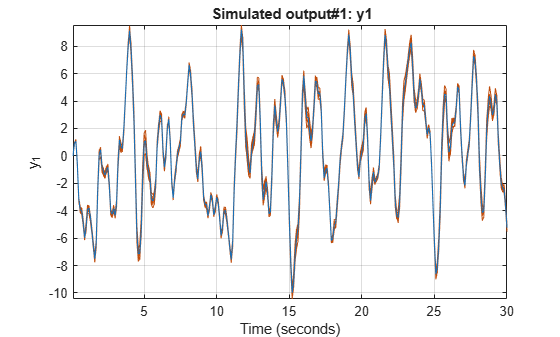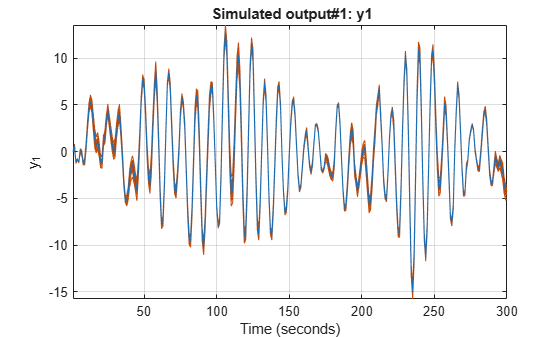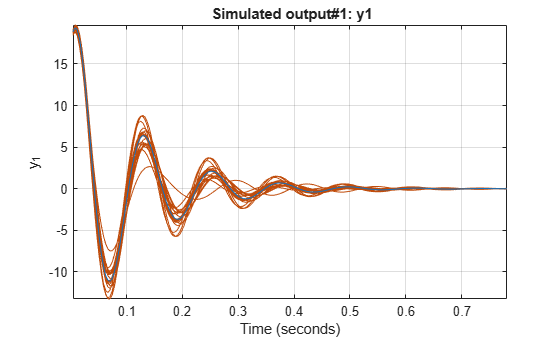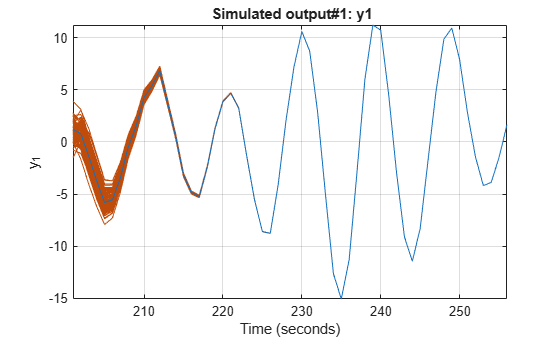Main Content

# simsd

Simulate linear models with uncertainty using Monte Carlo method

## Syntax

``simsd(sys,udata)``
``simsd(sys,udata,N)``
``simsd(sys,udata,N,opt)``
``y = simsd(___)``
``````[y,y_sd] = simsd(___)``````

## Description

`simsd` simulates linear models using the Monte Carlo method. The command performs multiple simulations using different values of the uncertain parameters of the model, and different realizations of additive noise and simulation initial conditions. `simsd` uses Monte Carlo techniques to generate response uncertainty, whereas `sim` generates the uncertainty using the Gauss Approximation Formula.

example

````simsd(sys,udata)` simulates and plots the response of 10 perturbed realizations of the identified model `sys`. Simulation input data `udata` is used to compute the simulated response.The parameters of the perturbed realizations of `sys` are consistent with the parameter covariance of the original model, `sys`. If `sys` does not contain parameter covariance information, the 10 simulated responses are identical. For information about how the parameter covariance information is used to generate the perturbed models, see Generating Perturbations of Identified Model. ```

example

````simsd(sys,udata,N)` simulates and plots the response of `N` perturbed realizations of the identified model `sys`.```

example

````simsd(sys,udata,N,opt)` simulates the system response using the simulation behavior specified in the option set `opt`. Use `opt` to specify uncertainties in the initial conditions and include the effect of additive disturbances.The simulated responses are all identical if `sys` does not contain parameter covariance information, and you do not specify additive noise or covariance values for initial states. You specify these values in the `AddNoise` and `X0Covariance` options of `opt`.```

example

````y = simsd(___)` returns the `N` simulation results in `y` as a cell array. No simulated response plot is produced. Use with any of the input argument combinations in the previous syntaxes.```

example

``````[y,y_sd] = simsd(___)``` also returns the estimated standard deviation `y_sd` for the simulated response.```

## Examples

collapse all

Load the estimation data.

`load iddata1 z1`

`z1` is an `iddata` object that stores the input-output estimation data.

Estimate a third-order state-space model.

`sys = ssest(z1,3);`

Simulate the response of the estimated model using the Monte Carlo method and input estimation data, and plot the response.

`simsd(sys,z1);`The blue line plots the simulated response of the original nominal model `sys`. The green lines plot the simulated response of 10 perturbed realizations of `sys`.

Simulate an estimated model using the Monte Carlo method for a specified number of model perturbations.

Estimate a second-order state-space model using estimation data. Obtain `sys` in the observability canonical form.

```load iddata3 z3 sys = ssest(z3,2,'Form','canonical');```

Compute the simulated response of the estimated model using the Monte Carlo method, and plot the responses. Specify the number of random model perturbations as 20.

```N = 20; simsd(sys,z3,N)```The blue line plots the simulated response of the original nominal model `sys`. The green lines plot the simulated response of the 20 perturbed realizations of `sys`.

You can also obtain the simulated response for each perturbation of `sys`. No plot is generated when you use this syntax.

`y = simsd(sys,z3,N);`

`y` is the simulated response, returned as a cell array of `N`+1 elements. `y{1}` contains the nominal response for `sys`. The remaining elements contain the simulated response for the `N` perturbed realizations.

Load time series data.

`load iddata9 z9`

`z9` is an `iddata` object with 200 output data samples and no inputs.

Estimate a sixth-order AR model using the least-squares algorithm.

`sys = ar(z9,6,'ls');`

For time series data, specify the desired simulation length, `Ns` = 200 using an `Ns`-by-0 input data set.

`data = iddata([],zeros(200,0),z9.Ts);`

Set the initial conditions to use the initial samples of the time series as historical output samples. The past data is mapped to the initial states of each perturbed system individually.

```IC = struct('Input',[],'Output',z9.y(1:6)); opt = simsdOptions('InitialCondition',IC);```

Simulate the model using Monte Carlo method and specified initial conditions. Specify the number of random model perturbations as 20.

`simsd(sys,data,20,opt)`The blue line plots the simulated response of the original nominal model `sys`. The green lines plot the simulated response of the 20 perturbed realizations of `sys`.

Load data, and split it into estimation and simulation data.

```load iddata3 ze = z3(1:200); zsim = z3(201:256);```

Estimate a second-order state-space model `sys` using estimation data. Specify that no parameter covariance data is generated. Obtain `sys` in the observability canonical form.

```opt = ssestOptions('EstimateCovariance',false); sys = ssest(ze,2,'Form','canonical',opt);```

Set the initial conditions for simulating the estimated model. Specify initial state values `x0` for the two states and also the covariance of initial state values `x0Cov`. The covariance is specified as a 2-by-2 matrix because there are two states.

```x0 = [1.2; -2.4]; x0Cov = [0.86 -0.39; -0.39 1.42]; opt = simsdOptions('InitialCondition',x0,'X0Covariance',x0Cov);```

Simulate the model using Monte Carlo method and specified initial conditions. Specify the number of random model perturbations as 100.

`simsd(sys,zsim,100,opt)`The blue line plots the simulated response of the original nominal model `sys`. The green lines plot the simulated response of the 100 perturbed realizations of `sys`. The software uses a different realization of the initial states to simulate each perturbed model. Initial states are drawn from a Gaussian distribution with mean `InitialCondition` and covariance `X0Covariance`.

Load the estimation data.

`load iddata1 z1`

`z1` is an `idddata` object that stores 300 input-output estimation data samples.

Estimate a second-order state-space model using the estimation data.

`sys = ssest(z1,2);`

Create a default option set for `simsd`, and modify the option set to add noise.

```opt = simsdOptions; opt.AddNoise = true;```

Compute the simulated response of the estimated model using the Monte Carlo method. Specify the number of random model perturbations as 20, and simulate the model using the specified option set.

`[y,y_sd] = simsd(sys,z1,20,opt);`

`y` is the simulated response, returned as a cell array of 21 elements. `y{1}` contains the nominal, noise-free response for `sys`. The remaining elements contain the simulated response for the 20 perturbed realizations of `sys` with additive disturbances added to each response.

`y_sd` is the estimated standard deviation of simulated response, returned as an `iddata` object with no inputs. The standard deviations are computed from the 21 simulated outputs. To access the standard deviation, use `y_sd.OutputData`.

## Input Arguments

collapse all

Model to be simulated, specified as one of the following parametric linear identified models: `idtf`, `idproc`, `idpoly`, `idss`, or `idgrey`.

To generate the set of simulated responses, the software perturbs the parameters of `sys` in a way that is consistent with the parameter covariance information. Use `getcov` to examine the parameter uncertainty for `sys`. For information about how the perturbed models are generated from `sys`, see `rsample`.

The simulated responses are all identical if `sys` does not contain parameter covariance information and you do not specify additive noise or covariance values for initial states. You specify these values in the `AddNoise` and `X0Covariance` options of `opt`.

Simulation input data, specified as one of the following:

• `iddata` object — Input data can be either time-domain or frequency-domain. The software uses only the input channels of the `iddata` object.

If `sys` is a time series model, that is, a model with no inputs, specify `udata` as an Ns-by-0 signal, where Ns is the wanted number of simulation output samples for each of the `N` perturbed realizations of `sys`. For example, to simulate 100 output samples, specify `udata` as follows.

`udata = iddata([],zeros(100,0),Ts);`

For an example, see Simulate Time Series Model Using Monte Carlo Method.

• matrix — For simulation of discrete-time systems using time-domain data only. Columns of the matrix correspond to each input channel.

If you do not have data from an experiment, use idinput to generate signals with various characteristics.

Number of perturbed realizations of `sys` to be simulated, specified as a positive integer.

Simulation options for simulating models using Monte Carlo methods, specified as a `simsdOptions` option set. You can use this option set to specify:

• Input and output signal offsets — Specify an offset to remove from the input signal and an offset to add to the response of `sys`.

• Initial condition handling — Specify initial conditions for simulation and their covariance. For state-space and linear grey-box models (`idss` and `idgrey`), if you want to simulate the effect of uncertainty in initial states, set the `InitialCondition` option to a double vector, and specify its covariance using the `X0Covariance` option. For an example, see Study Effect of Initial Condition Uncertainty on Model Response.

• Addition of noise to simulated data — If you want to include the influence of additive disturbances, specify the `AddNoise` option as `true`. For an example, see Study Effect of Additive Disturbance on Response Uncertainty.

## Output Arguments

collapse all

Simulated response, returned as a cell array of `N`+1 elements. `y{1}` contains the nominal response for `sys`. The remaining elements contain the simulated response for the `N` perturbed realizations.

The command performs multiple simulations using different values of the uncertain parameters of the model, and different realizations of additive noise and simulation initial conditions. Thus, the simulated responses are all identical if `sys` does not contain parameter covariance information and you do not specify additive noise and covariance values for initial states in `opt`.

Estimated standard deviation of simulated response, returned as an `iddata` object. The standard deviation is computed as the sample standard deviation of the y ensemble:

`$y_sd=\sqrt{\frac{1}{N}\sum _{i=2}^{N+1}{\left(y\left\{1\right\}-y\left\{i\right\}\right)}^{2}}$`

Here `y{1}` is the nominal response for `sys`, and `y{i}` (```i = 2:N+1```) are the simulated responses for the `N` perturbed realizations of `sys`.

## More About

collapse all

### Generating Perturbations of Identified Model

The software generates `N` perturbations of the identified model `sys` and then simulates the response of each of these perturbations. The parameters of the perturbed realizations of `sys` are consistent with the parameter covariance of the original model `sys`. The parameter covariance of `sys` gives information about the distribution of the parameters. However, for some parameter values, the resulting perturbed systems can be unstable. To reduce the probability of generation of unrealistic systems, the software prescales the parameter covariance.

If Δp is the parameter covariance for the parameters p of `sys`, then the simulated output f(p+Δp) of a perturbed model as a first-order approximation is:

`$f\left(p+\Delta p\right)=f\left(p\right)+\frac{\partial f}{\partial p}\Delta p$`

The `simsd` command first scales Δp by a scaling factor s (approximately 0.1%) to generate perturbed systems with parameters (p+sΔp). The command then computes f(p+sΔp), the simulated response of these perturbed systems. Where,

`$f\left(p+s\Delta p\right)=f\left(p\right)+s\frac{\partial f}{\partial p}\Delta p$`

The command then computes the simulated response f(p+Δp) as:

`$f\left(p+\Delta p\right)=f\left(p\right)+\frac{1}{s}\left(f\left(p+s\Delta p\right)-f\left(p\right)\right)$`

Note

This scaling is not applied to the free delays of `idproc` or `idtf` models.

If you specify the `AddNoise` option of `simsdOptions` as `true`, the software adds different realizations of the noise sequence to the noise-free responses of the perturbed system. The realizations of the noise sequence are consistent with the noise component of the model.

For state-space models, if you specify the covariance of initial state values in `X0Covariance` option of `simsdOptions`, different realizations of the initial states are used to simulate each perturbed model. Initial states are drawn from a Gaussian distribution with mean `InitialCondition` and covariance `X0Covariance`.

## See Also

Introduced before R2006a

## SupportGet trial now